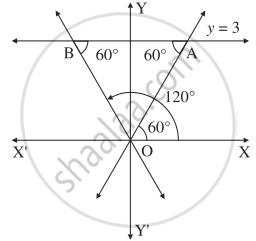Advertisement Remove all ads

# Find the combined equation of the pair of lines through the origin and making an equilateral triangle with the line y = 3. - Mathematics and Statistics

Sum

Find the combined equation of the pair of lines through the origin and making an equilateral triangle with the line y = 3.

Advertisement Remove all ads

#### Solution

Let OA and OB be the lines through the origin making an angle of 60° with the line y = 3.

∴ OA and OB make an angle of 60° and 120° with the positive direction of the X-axis.

∴ slope of OA = tan 60° = sqrt3

∴ equation of the line OA is

y = sqrt3x i.e. sqrt3x - y = 0Slope of OB = tan 120° = tan (180° - 60°)

= - tan 60° = - sqrt3

∴ equation of the line OB is

y = - sqrt3x, i.e. sqrt3x + y = 0

∴ required joint equation of the lines is

(sqrt3"x" - "y")(sqrt3"x" + "y") = 0

i.e. 3x2 - y2 = 0

Concept: Homogeneous Equation of Degree Two
Is there an error in this question or solution?
Advertisement Remove all ads

#### APPEARS IN

Advertisement Remove all ads
Advertisement Remove all ads
Share
Notifications

View all notifications

Forgot password?